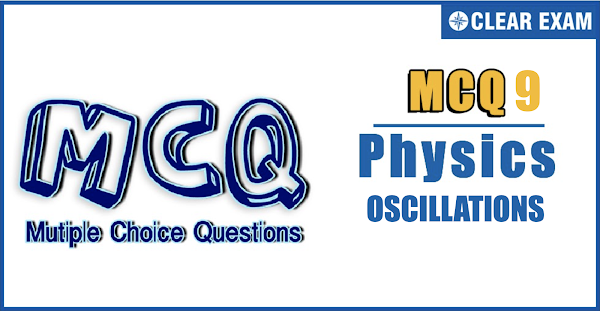## [LATEST]\$type=sticky\$show=home\$rm=0\$va=0\$count=4\$va=0

JEE Advanced Physics Syllabus can be referred by the IIT aspirants to get a detailed list of all topics that are important in cracking the entrance examination. JEE Advanced syllabus for Physics has been designed in such a way that it offers very practical and application-based learning to further make it easier for students to understand every concept or topic by correlating it with day-to-day experiences. In comparison to the other two subjects, the syllabus of JEE Advanced for physics is developed in such a way so as to test the deep understanding and application of concepts..

Q1. A block of mass 4 kg hangs from a spring of spring constant k=400 N/m. The block is pulled down through 15 cm below and released. What is its kinetic energy when the block is 10 cm above the equilibrium position?
•  a) 5J
•  b) 2.5J
•  c) 1J
•  d) 1.9J
Q2.The displacement y of a particle executing periodic motion is given by y=4 cos2(t/2) sin⁡(1000t). This expression may be considered to be a result of the superposition of…….. independent harmonic motions
•  Two
•  There
•  Four
•  Five
Q3.  A simple pendulum has time period T1. The point of suspension is now moved upward according to the relation y=k t2,(k=1 ms(-2) ) where y is the vertical displacement. The time period now becomes T2. The ratio of (T12)/(T22 ) is (g=10 ms(-2) )
•  6/5
•  5/6
•  1
•  4/5

Q4. A simple pendulum is making oscillations with its bob immersed in a liquid of density n times less than the density of the bob. What is its period?
•  a) 2π√(l/ng)
•  b) 2π√(l/(1-1/n)g)
•  c) 2π√(ln/g)
•  d) 2π√(l/(n-1)g)
Q5.One end of a long metallic wire of length L is tied to the ceiling. The other end is tied to massless spring of spring constant K. A mass m hangs freely from the free end of the spring. The area of cross-section and Young’s modulus of the wire are A and Y respectively. If the mass is slightly pulled down and released, it will oscillate with a time period T equal to
•  2π(m/K)
•  2π{(YA+KL)m/YAK}1/2
•  2π mYA/KL
•  2π mL/YA
Q6. An object of mass 4 kg is attached to a spring having spring constant 100 N/m. It performs simple harmonic motion on a smooth horizontal surface with an amplitude of 2m. A 6 kg object is dropped vertically onto the 4 kg object when it crosses the mean position, and sticks to it. The change in amplitude of oscillation due to collision is
•  1 m
•  Zero
•  2[1-√(2/5)]
•  2[1-1/√5]

Q7.Two particles are executing identical simple harmonic motions described by the equations, x1=〖a cos〗⁡〖(ωt+(π/6))〗 and x2=〖a cos〗⁡〖(ωt+π/3〗). The minimum interval of time between the particles crossing the respective mean positions is
•  π/2ω
•  π/3ω
•  π/4ω
•  π/6ω
Q8.Two simple harmonic motions are represented by equations y1=4 sin⁡(10t+ϕ) y2=5 cos⁡10t What is the phase difference between their velocities?
•  ϕ
•
•  (ϕ+π/2)
•  (ϕ-π/2)
Q9.The mass and diameter of a planet are twice those of earth. The period of oscillation of pendulum on this planet will be (If it is a second’s pendulum on earth)
•  1/√2 s
•  2√2 s
•  2 s
•  1/2 s
Q10. A simple pendulum of length l and a mass m of the bob is suspended in a car that is travelling with a constant speed v around a circle of radius R. If the pendulum undergoes small oscillations about its equilibrium position, the frequency of its oscillation will be
•  1/2π √(g/l)
•  1/2π √(g/R)
•  1/2π √((g2+v4/R2 )(1/2)/l)
•  1/2π √(v2/Rl)#### Written by: AUTHORNAME

AUTHORDESCRIPTION## Want to know more

Please fill in the details below:

## Latest NEET Articles\$type=three\$c=3\$author=hide\$comment=hide\$rm=hide\$date=hide\$snippet=hide

Name

ltr
item
BEST NEET COACHING CENTER | BEST IIT JEE COACHING INSTITUTE | BEST NEET, IIT JEE COACHING INSTITUTE: Oscillations-Quiz-9
Oscillations-Quiz-9
https://1.bp.blogspot.com/-WtLbut5SO3A/YOXr-pkRUkI/AAAAAAAAgUY/d5ztZOesA-cbpeFcuhj6unGddck0Y2NswCLcBGAsYHQ/s600/Screenshot%2B2021-07-04%2Bat%2B3.00.49%2BPM.png
https://1.bp.blogspot.com/-WtLbut5SO3A/YOXr-pkRUkI/AAAAAAAAgUY/d5ztZOesA-cbpeFcuhj6unGddck0Y2NswCLcBGAsYHQ/s72-c/Screenshot%2B2021-07-04%2Bat%2B3.00.49%2BPM.png
BEST NEET COACHING CENTER | BEST IIT JEE COACHING INSTITUTE | BEST NEET, IIT JEE COACHING INSTITUTE
https://www.cleariitmedical.com/2021/07/oscillations-quiz-9.html
https://www.cleariitmedical.com/
https://www.cleariitmedical.com/
https://www.cleariitmedical.com/2021/07/oscillations-quiz-9.html
true
7783647550433378923
UTF-8